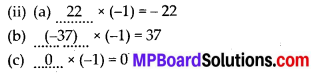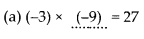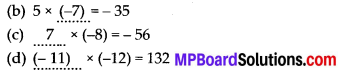# MP Board Class 7th Maths Solutions Chapter 1 Integers Ex 1.3

## MP Board Class 7th Maths Solutions Chapter 1 Integers Ex 1.3

Question 1.
Find each of the following products:
(a) 3 × (-1)
(b) (-1) × 225
(c) (-21) × (-30)
(d) (-316) × (-1)
(e) (-15) × 0 × (-18)
(f) (-12) × (-11) × (10)
(g) 9 × (-3) × (- 6)
(h) (-18) × (-5) × (-4)
(i) (-1) × (-2) × (-3) × 4
(j) (-3) × (-6) × (-2) × (-1)
Solution:
(a) 3 × (-1) = _ 3
(b) (-1) × 225 = – 225
(c) (-21) × (-30) = 630 .
(d) (-316) × (-1) = 316
(e) (-15) × 0 × (-18) = 0
(f) (-12) × (-11) × 10 = 1320
(g) 9 × (-3) × (-6) = 162
(h) (-18) × (-5) × (-4) = -360
(i) (-1) × (-2) × (-3) × 4 = -24
(j) (-3) × (-6) × (-2) × (-1) = 36Question 2.
Verify the following:
(a) 18 × [7 + (-3)] = [18 × 7] + [18 × (-3)]
(b) (-21) × [(- 4) + (- 6)]
= [(-21) × (- 4)] + [(-21) × (- 6)]
Solution:
(a) L.H.S. = 18 × [7 + (-3)]
= 18 × [7 – 3] = 18 × 4 = 72
R.H.S. = [18 × 7] + [18 × (-3)] = 126 – 54 = 72
Hence, 18 × [7 + (-3)] = [18 × 7] + [18 × (-3)]
= [(-21) × (-4)] + [(-21) × (-6)]

Question 3.
(i) For any integer a, what is (-1) × a equal to?
(ii) Determine the integer whose product with (-1) is
(a) -22
(b) 37
(c) 0
Solution:
(i) (-1) × a = -aQuestion 4.
Starting from (-1) × 5, write various products showing some pattern to show (-1) × (-1) = 1.
Solution:
-1 × 5 = – 5
-1 × 4 = -4 = – 5 + 1; -1 × 3 = -3 = -4 + 1
-1 × 2 = -2 = -3 + 1; -1 × 1= -1 = -2 + 1
-1 × 0 = 0 = – 1 + 1;
Therefore, -1 × (-1) = 0 + 1 = 1Question 5.
Find the product using suitable properties:
(a) 26 × (- 48) + (- 48) × (-36)
(b) 8 × 53 × (-125)
(c) 15 × (-25) × (- 4) × (-10)
(d) (-41) × 102
(e) 625 × (-35) + (- 625) × 65
(f) 7 × (50 – 2)
(g) (-17) × (-29)
(h) (-57) × (-19)+ 57
Solution:
(a) 26 × (-48) + (-48) × (-36)
= (-48) × 26 + (-48) × (-36) (∵ b × a =a × b)
= (-48)[26 – 36] [∵ (a × b) + (a × c) = a × (b + c)] = (-48) × (-10) = 480

(b) 8 × 53 × (-125) = 8 × [53 × (-125)]
[∵ a × b × C = a × (b × C)] = 8 × (- 6625) = – 53000

(c) 15 × (-25) × (-4) × (-10)
= 15 × [(-25) × (-4)] × (-10)
= 15 ×  × (-10) = 15 × (-1000)
= -15000

(d) (-41) × 102 = (-41) × (100 + 2)
= (-41) × 100 + (-41) × 2
[∵ a × (b + c) = (a × b) + (a × c)]
= -4100 – 82 = -4182

(e) 625 × (-35) + (-625) × 65
= 625 × [(-35) + (-65)]
(a × b) + (a × c) = a × (b + c)]
= 625 × [-100] = – 62500

(f) 7 × (50 – 2) = (7 × 50) – (7 × 2)
[ ∵ a × (b – c) = (a × b) – (a × c)]
= 350 – 14 = 336

(g) (-17) × (-29) = (-17) × [-30 + 1]
= [(-17) × (-30)] + [(-17) × 1]
[∵ a × (b + c) = (a × b) + (a × c)] =  + [-17] = 493

(h) (-57) × (-19) + 57 = (57 × 19) + (57 × 1)
= 57 × [19 +1]
[∵ (a × b) + (a × c) = a × (b + c)]
= 57 × 20
= 1140Question 6.
A certain freezing process requires that room temperature be lowered from 40°C at the rate of 5°C every hour. What will be the room temperature 10 hours after the process begins?
Solution:
Initial temperature = 40°C
Change in temperature per hour = – 5°C
Change in temperature after 10 hours = (-5) × 10 = – 50°C
Hence, final temperature = 40°C + (-50°C)
= -10°C

Question 7.
In a class test containing 10 questions, 5 marks are awarded for every correct answer and (-2) marks are awarded for every incorrect answer and 0 for questions not attempted.
(i) Mohan gets four correct and six incorrect answers. What is his score?
(ii) Reshma gets five correct answers and five incorrect answers, what is her score?
(iii) Heena gets two correct and five incorrect answers out of seven questions she attempts. What is her score?
Solution:
Marks given for 1 correct answer = 5
Marks given for 1 wrong answer = – 2
(i) Marks given for 4 correct answers = 5 × 4 = 20
Marks given for 6 wrong answers = -2 × 6 = -12
Marks obtained by Mohan = 20 – 12 = 8

(ii) Marks given for 5 correct answers = 5 × 5 = 25
Marks given for 5 wrong answers = -2 × 5 = – 10
Marks obtained by Reshma = 25 – 10 = 15

(iii) Marks given for 2 correct answers = 5 × 2 = 10
Marks given for 5 wrong answers = -2 × 5 = -10
∴ Marks obtained by Heena = 10 – 10 = 0Question 8.
A cement company earns a profit of ₹ 8 per bag of white cement sold and a loss of ₹ 5 per bag of grey cement sold.
(a) The company sells 3,000 bags of white cement and 5,000 bags of grey cement in a month. What is its profit or loss?
(b) What is the number of white cement bags it must sell to have neither profit nor loss, if the number of grey bags sold is 6,400 bags.
Solution:
Profit is denoted by a positive integer and loss is denoted by a negative integer.
Profit earned while selling 1 bag of white cement = ₹ 8
Loss incurred while selling 1 bag of grey cement = – ₹ 5
(a) Profit earned while selling 3000 bags of white cement = ₹ 8 × 3000 = ₹ 24000
Loss incurred while selling 5000 bags of grey cement = ₹ (5 × 5000) = -₹ 25000
Total profit/loss earned = Profit + Loss
= ₹ [24000 + (-25000)] = – ₹ 1000
Therefore, a loss of ₹ 1000 will be incurred by the company.

(b) Loss incurred while selling 6400 bags of grey cement = ₹ (-5) × 6400 = – ₹ 32000
Let x number of bags of white cement to be sold.
Profit earned while selling x bags of white cement = ₹ x × 8 = ₹ 8,
In condition of no profit no loss,
Profit earned + Loss incurred = 0
8x + (-32000) = 0 ⇒ 8x = 32000 ⇒ x = 4000
Therefore, 4000 bags of white cement must be sold.Question 9.
Replace the blank with an integer to make it a true statement.
(a) (-3) × ….. = 27
(b) 5 × ….. = -35
(c) …… × (-8) = -56
(d) …… × (-12) = 132
Solution: